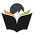# Data Structures and Algorithm Analysis Set 18

## Data Structures and Algorithm Analysis

### Questions 171 to 180

171.
Consider the following Traveling salesperson problem instance matrix. What would be the value of g(1, {2, 3})?
 ∞ 8 5 1 ∞ 7 2 3 ∞

 (a) 2 (b) 12 (c) 14 (d) 17 (e) 9
172.
The running time complexity of graph coloring problem is
 (a) O(nm) (b) O(mn) (c) O(mnm) (d) O(nmn) (e) O(mnnm).
173.
What is the time taken by the binary search algorithm to search a key ‘k’ in a sorted array of ‘n’ elements?
 (a) O(log2 n) (b) O(n) (c) (n log2 n) (d) O(n2) (e) O(1).
174.
Name the function which is used to kill live nodes without generating all their children in state space tree using backtracking method
 (a) Solution function (b) Bounding function (c) Optimal function (d) State Space function (e) Randomized function.
175.
Which of the following search method will always find a goal node nearest to the root of the tree?
 (a) Depth first search (b) Binary search (c) Breadth first search (d) Heuristic search (e) Randomized search.
176.
What do we call the selected keys in quick sort?
 (a) Recombine key (b) Inner key (c) Outer key (d) Pivot key (e) Median key.
177.
This sort finds location ‘pos’ of smallest elements in a(i), …. , a(n) and then interchange a(pos) with a(i) for i = 1, …., n – 1.
 (a) Selection sort (b) Quick sort (c) Heap sort (d) Bubble sort (e) Insertion sort.
178.
Adjacency matrix of a digraph is
 (a) Identity matrix (b) Symmetric matrix (c) Asymmetric matrix (d) Sparse matrix (e) Zero matrix.
179.
We can efficiently reverse a string using
 (a) Linear queue (b) Stack (c) Circular queue (d) Doubly linked list (e) Circular linked list.
180.
The technique of linear probing for collision resolution can lead to
 (a) Underflow (b) Radix ordering (c) Efficient storage utilization (d) Overflow (e) Clustering.

#### Answers

 171 Answer  :        (e) Reason : This is  g( 1, {2,3}) = min( C12 + g( 2, {3}), C13 + g( 3, {2}) = min( 8+9, 5+4 ) = 9. 172 Answer : (d) Reason:  According to the complexity analysis of the algorithm. 173 Answer : (a) Reason:  According to the mathematical analysis binary search takes ( log2 n ) time. 174 Answer : (b) Reason:  Because, in state space tree generation of backtracking approach a bounding function is used to kill live nodes without generating all their children, if that node is not promising the solution. 175 Answer : (c) Reason:  Because in this method nodes are generated level by level and hence the goal node is found nearest to root node. 176 Answer : (d) Reason:  Because, in quick sort division is made along the pivotal element. 177 Answer : (a) Reason:  As this process is the key process for the selection sort algorithm. 178 Answer : (c) Reason:  Because, in a diagraph for directed edge ; b is adjacent to a, but reverse is not true. Hence the adjacency matrix is asymmetric matrix. 179 Answer : (b) Reason:  This is nothing but pushing the characters of the string in a stack and then popping the stack to get the reversed order of the string. 180 Answer : (e) Reason:  Because, if we follow the linear probing method to resolve the collision then it may lead to the clustering.

<< PrevNext >>

#### 2 comments :

1.2.